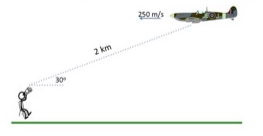# Problem: You are standing in a field and see a 4000 kg airplane flying at horizontally at 250 m/s. The distance from you to the airplane is 2km, along a line 30° from the horizontal. The figure shows the airplane, flying from right to left of the page. What is the magnitude and direction of the angular momentum of the airplane with respect to you, the observer? a) 106 kg m/s b) 107 kg m/s c) 109 kg m/s d) 108 kg m/s e) 2 x 106 kg m/s

###### FREE Expert Solution

The angular momentum is:

$\overline{)\begin{array}{rcl}{\mathbf{L}}& {\mathbf{=}}& \mathbf{r}\mathbf{×}\mathbf{m}\mathbf{v}\end{array}}$

r = [(2)cos(30°)i + (2)sin(30°)j]km

r = (1.73205i + j)km = (1732.05i + 1000j) m

79% (207 ratings)###### Problem Details

You are standing in a field and see a 4000 kg airplane flying at horizontally at 250 m/s. The distance from you to the airplane is 2km, along a line 30° from the horizontal. The figure shows the airplane, flying from right to left of the page.What is the magnitude and direction of the angular momentum of the airplane with respect to you, the observer?

a) 106 kg m/s

b) 107 kg m/s

c) 109 kg m/s

d) 108 kg m/s

e) 2 x 106 kg m/s

Frequently Asked Questions

What scientific concept do you need to know in order to solve this problem?

Our tutors have indicated that to solve this problem you will need to apply the Angular Momentum of Objects in Linear Motion concept. You can view video lessons to learn Angular Momentum of Objects in Linear Motion. Or if you need more Angular Momentum of Objects in Linear Motion practice, you can also practice Angular Momentum of Objects in Linear Motion practice problems.